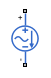# SFFM Current Source

Single-frequency frequency-modulated current source

•Libraries:
Simscape / Electrical / Additional Components / SPICE Sources

## Description

The SFFM Current Source block represents a frequency-modulated AC current source whose output current value is independent of the voltage across its terminals. The following equation describes the current through the source as a function of time:

`${I}_{out}=IO+IA*\mathrm{sin}\left(\left(2\pi *FC*Time\right)+MI*\mathrm{sin}\left(2\pi *FS*Time\right)\right)$`

where:

• I0 is the Current offset, IO parameter value.

• IA is the Current amplitude, IA parameter value.

• FC is the Carrier frequency, FC parameter value.

• MI is the Modulation index, MI parameter value.

• FS is the Signal frequency, FS parameter value.

The block uses a small conductance internally to prevent numerical simulation issues. The conductance connects the + and - ports of the device and has a conductance GMIN:

• By default, GMIN matches the GMIN parameter of the Environment Parameters block, whose default value is 1e–12.

• To change GMIN, add an Environment Parameters block to your model and set the GMIN parameter to the desired value.

## Ports

### Conserving

expand all

Electrical conserving port associated with the SFFM current source positive terminal.

Electrical conserving port associated with the SFFM current source negative terminal.

## Parameters

expand all

Magnitude of the time-independent part of the output current.

Magnitude of the sinusoidal part of the output current.

Frequency of the carrier wave. The value must be greater than or equal to `0`.

Amount by which the modulated signal varies around its unmodulated level. The value must be greater than or equal to `0`.

Frequency of the modulated signal. The value must be greater than or equal to `0`.

Specify if the current source includes a parallel conductance. The value of the parallel conductance, GMIN, depends on whether your model contains an Environment Parameters block.

This table shows the possible values of the parallel conductance, GMIN:

Values of parallel conductance, GMIN

Include parallel conductance, GMIN
`Yes``No`
Model contains Environment Parameters blockModel does not contain Environment Parameters blockParallel conductance, GMIN, is not included and it does not affect the current source.
GMIN is equal to the value of the GMIN parameter in the Environment Parameters block.GMIN = `1e-12` `Ohm`

## Version History

Introduced in R2008a